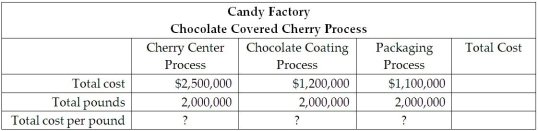/
/
/
31) The Candy Factory manufactures healthy candy that it sells
Not my Question
Flag Content

# Question : 31) The Candy Factory manufactures healthy candy that it sells : 1876970

31) The Candy Factory manufactures healthy candy that it sells to consumers and groups in themarketplace. The business produces chocolate-covered cherries in a three-step process to develop the center of the cherries, coat the cherries in chocolate, and package the final product. The total cost to produce a pound of chocolate covered cherries is the sum of the cost per pound in each of the following processes:The managerial accountant reported that there is no Work in Process Inventory. Assuming that there is no work in process inventories, calculate the total cost per pound for each of the three processes listed above and the total cost to produce a pound of chocolate covered cherries. Explain why the cost in each process is important to the managerial accountant. Discuss at least three reasons why the cost in the process is important and how this cost is used at the company.

32) In process costing, manufacturing costs are usually combined into two categories: direct materials and conversion costs.

33) Conversion costs are generally added evenly throughout a process.

34) Equivalent units express the amount of work done during a period in terms of partially completed units.

35) Equivalent units should be computed separately for direct materials and conversion costs.

36) The number of partially completed units multiplied by the percentage of process completed equals the number of equivalent units.

37) Equivalent units express the amount of work done during a period of time in terms of fully completed units of output.

38) If a company has 6,000 units that are 60% complete, the equivalent unit figure is 3,600.

39) Conversion costs are usually incurred evenly throughout productions.

40) In a process costing environment, direct materials and direct labor are usually combined into one cost category called "conversion costs."

## Solution 5 (1 Ratings )

Solved
Accounting 11 Months Ago 9 Views